# Introduction to Survival Analysis in R

UCLA Office of Advanced Research Computing
Statistical Methods and Data Analytics

# Purpose

This workshop aims to provide just enough background in survival analysis to be able to use the survival package in R to:

• estimate survival functions
• test whether survival functions are different between groups
• fit a Cox proportional hazards model

# Workshop packages

The survival package:

• provides all tools used in this workshop to estimate survival analysis models and tests
• created by Terry Therneau, researcher and expert in survival analysis, so package is trustworthy
• Therneau co-authored Modeling Survival Data: Extending the Cox Model with Patricia Grambsch, a reference book for survival analysis and the survival package
• Grambsch and Therneau developed some of the methods used to assess the proportional hazards assumption of the Cox model
• widely used, so has inspired many additional packages to extend its functionality, like survminer

We use the survminer for its ggsurvplot() function, used to create highly customizable plots of survival functions

We use the broom package for its tidy() function, which cleans up output tables and stores them as data frames.

If you are following in RStudio, go ahead and load the workshop packages now with library().

library(survival)
library(survminer) # for customizable graphs of survival function
library(broom) # for tidy output
library(ggplot2) # for graphing (actually loaded by survminer)

# Outline

1. Quick review of survival analysis
2. Setting up data for survival analysis
3. Kaplan-Meier estimator of the survival function
4. Comparing survival curves
5. Cox model introduction
6. Fitting a Cox model with coxph()
7. Predictions from A Cox model
8. Assessing the proportional hazards assumption
9. Time-varying covariates

# What is survival analysis?

Survival analysis models how much time elapses before an event occurs.

The outcome variable, the length of time to an event, is often referred to as either survival time, failure time, or time to event.

Example events include:

• death upon contracting a disease
• divorce
• malfunctioning of a machine
• first job

Events are often referred to as failures.

Almost anything can be framed as the event of interest, so survival analysis has broad applications across many fields.

We often say that the subject is at risk and a member of the risk set before the event occurs or the subject’s time is censored.

# Survival function

One of the goals of survival analysis is to estimate the probability that a subject survives without experiencing the event past some time $$t$$.

We can infer these probabilities from observing how long different subjects remain at risk before failing, i.e., observing their survival times.

Let $$T$$ be a random variable representing a subject’s true survival time.

Sometimes, we cannot observe a subject’s true survival time $$T$$ during the course of a study, known as censoring. In general, we say we observe subjects’ follow-up time, which for some will be the true survival time $$T$$ and for others will be the censoring time.

The survival function, $$S(t)$$ expresses the probability that a subject’s true survival time $$T$$ will exceed time $$t$$, i.e., that the subject will survive beyond time $$t$$.

$S(t)=Pr(T>t)$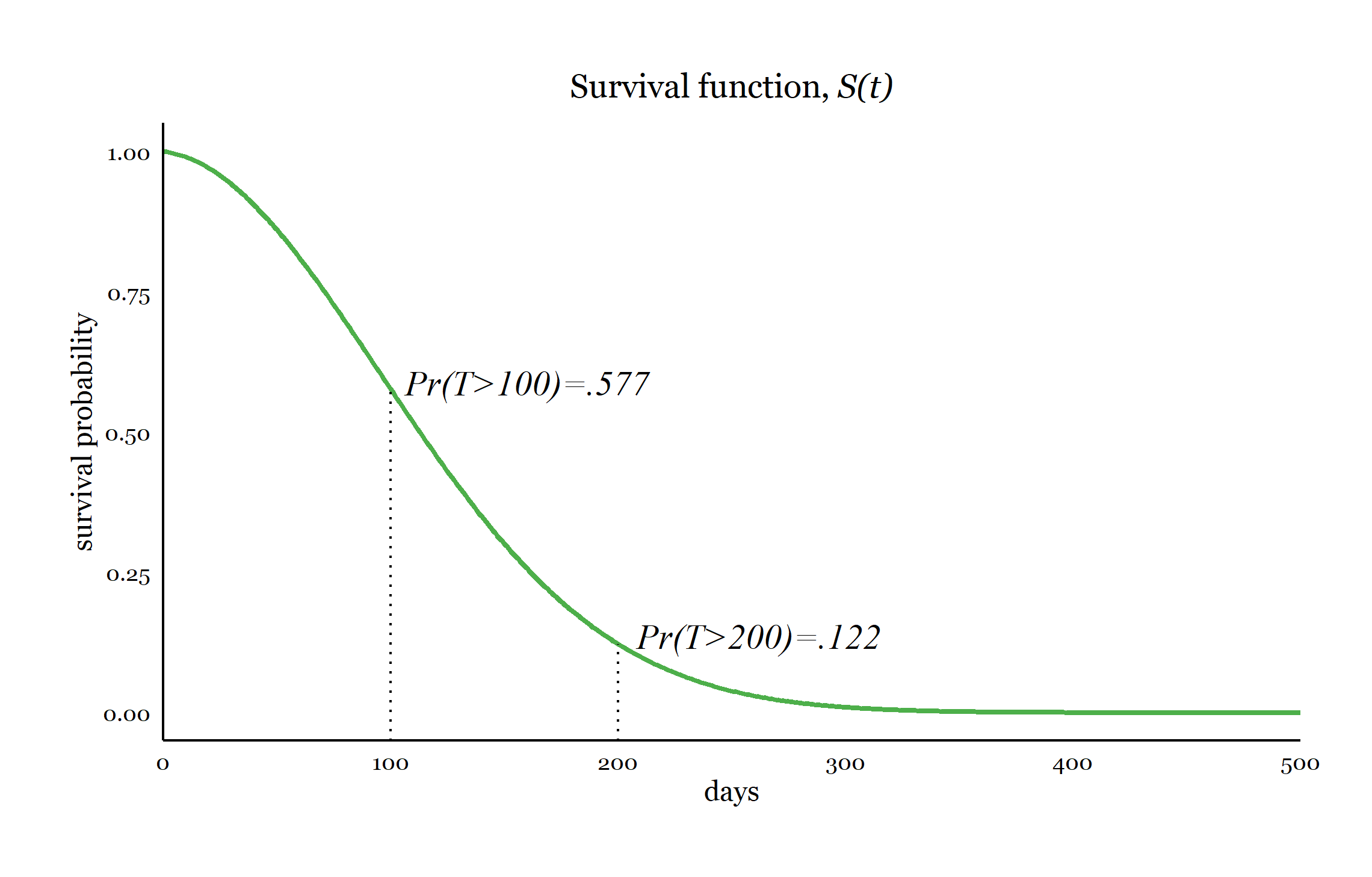For the survival curve above,

$$S(100)=.577$$, the probability that a subject survives beyond 100 days is 0.577.

$$S(200)=.122$$, the probability that a subject survives beyond 200 days is 0.122.

Typically, we also assume:

$$S(0)=1$$, all subjects survive the very first moment

$$S(\infty)=0$$, all subjects fail after infinite time

Median survival time is defined as the time $$t$$ at which 50% of the population is expected to be still surviving: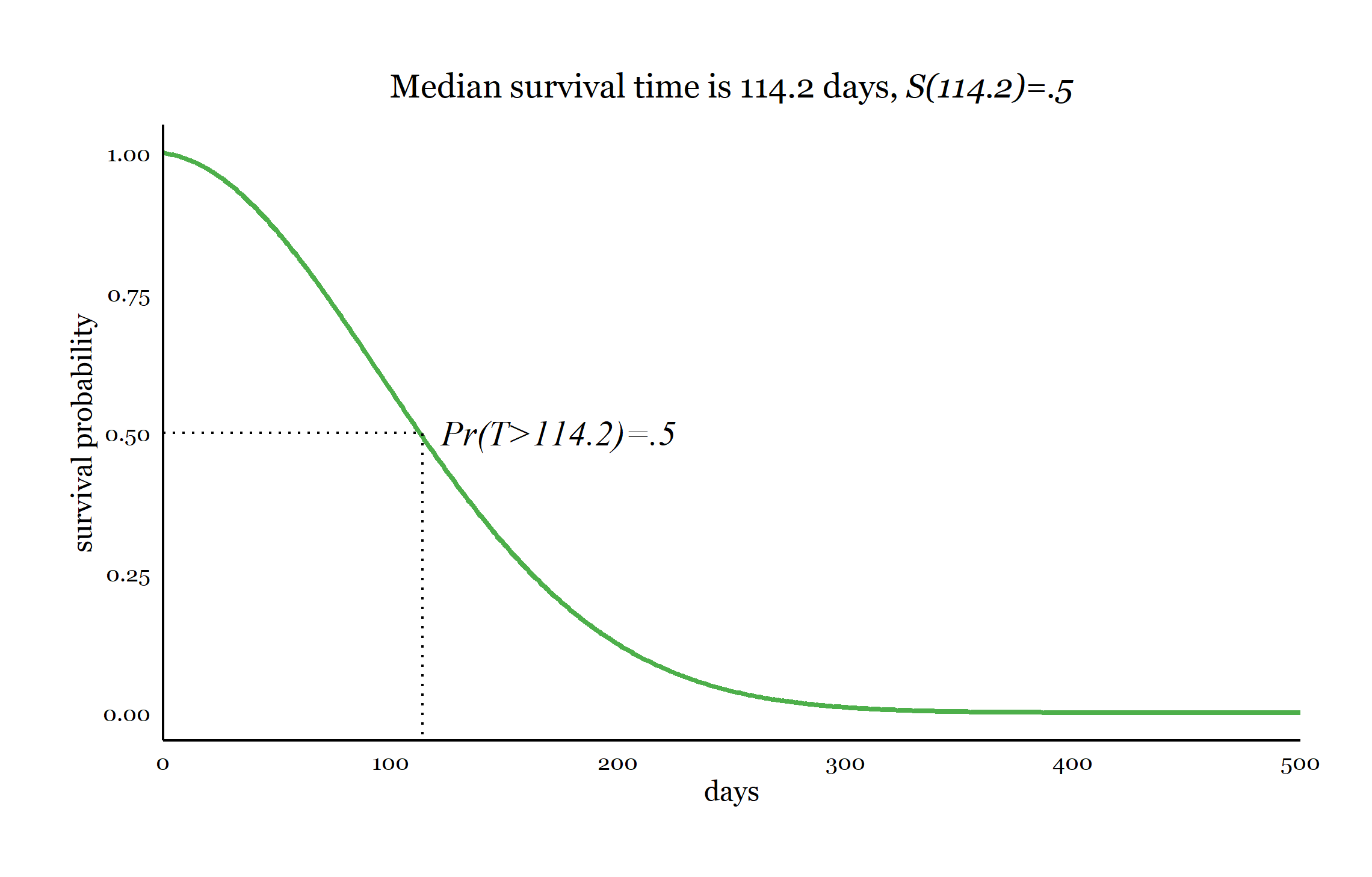# Hazard function

Some survival methods, such as the Kaplan-Meier estimator, focus on estimating the survival function $$S(t)$$ directly.

Other methods, such as the Cox model, focus on the hazard function (also known as the hazard rate), $$h(t)$$, which is inversely related to the $$S(t)$$.

The hazard function at time $$t$$, $$h(t)$$, is defined as the instantaneous rate of events at time $$t$$, given that the subject has survived until time $$t$$. We say instantaneous because $$h(t)$$ may be changing moment to moment, continuously over time.

For example, in the green curve below, $$h(200)=\frac{\text{.0204 events}}{day}$$, while $$h(200.1)=\frac{\text{.02041 events}}{day}$$.

With an increase in the hazard function, more events are expected per unit time, and survival will be expected to decrease.

Below we see three examples of hazard functions, 2 of which are changing continuously with time.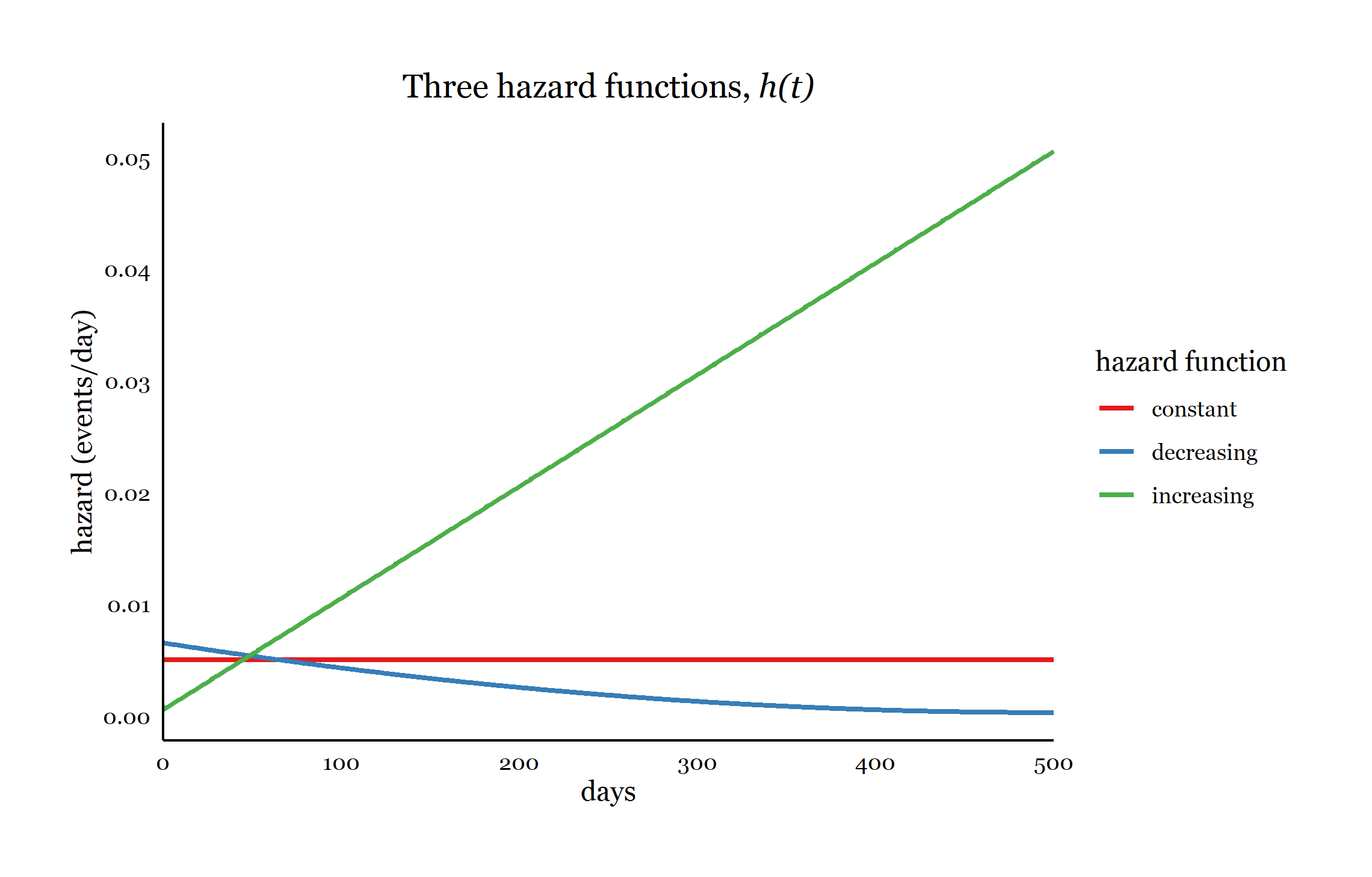Note: $$h(t)\ge0$$, so the hazard function can never be negative

# Cumulative hazard

The cumulative hazard function, $$H(t)$$, expresses how much hazard a subject has accumulated over time up to time $$t$$.

$H(t)=\int_0^t h(u)du$ The probability that a subject will fail over time increases as the hazard accumulates.

Because the hazard function $$h(t)$$ is never negative, the cumulative hazard $$H(t)$$ can never decrease with time.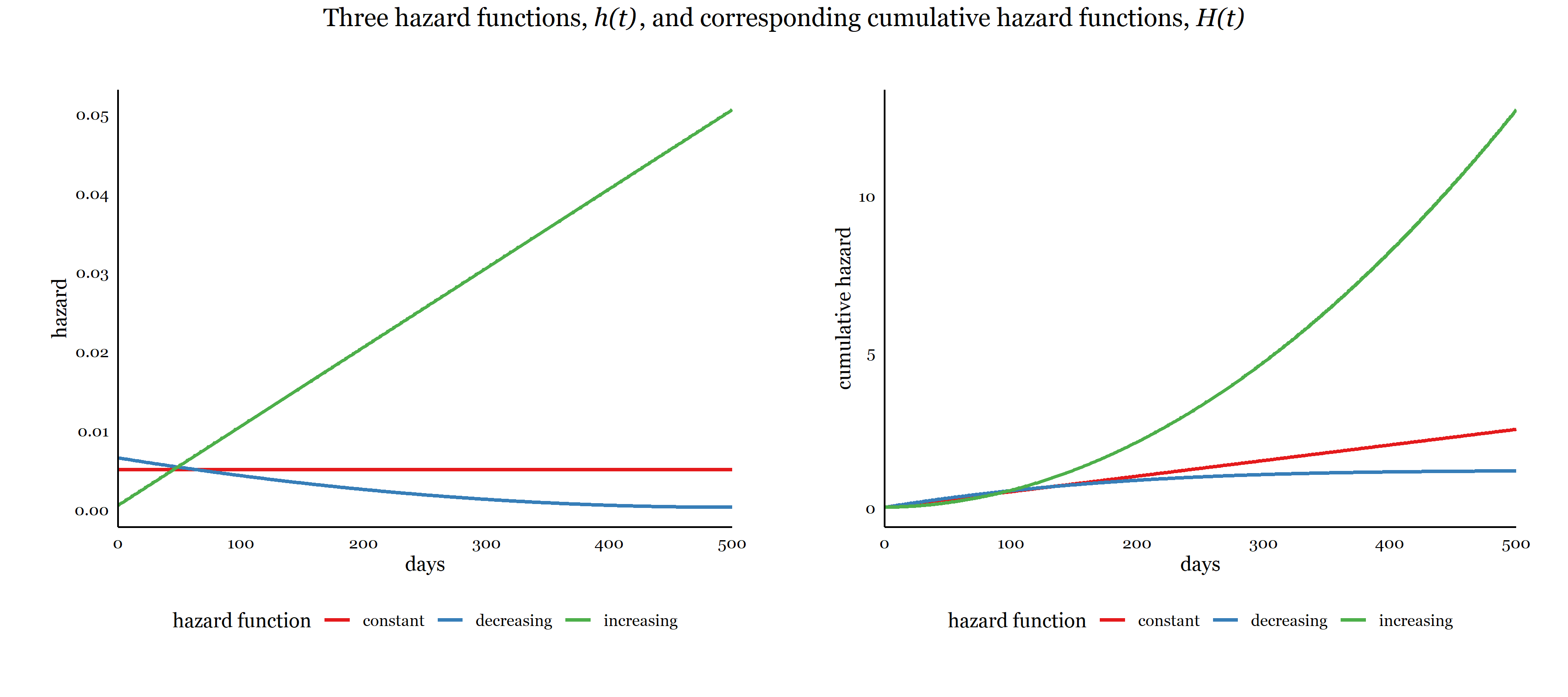# Relationship between the hazard and survival functions

The survival function is inversely related to the cumulative hazard function, where we see that as a subject’s cumulative hazard grows, the survival probability decreases.

$S(t)=exp(-H(t))$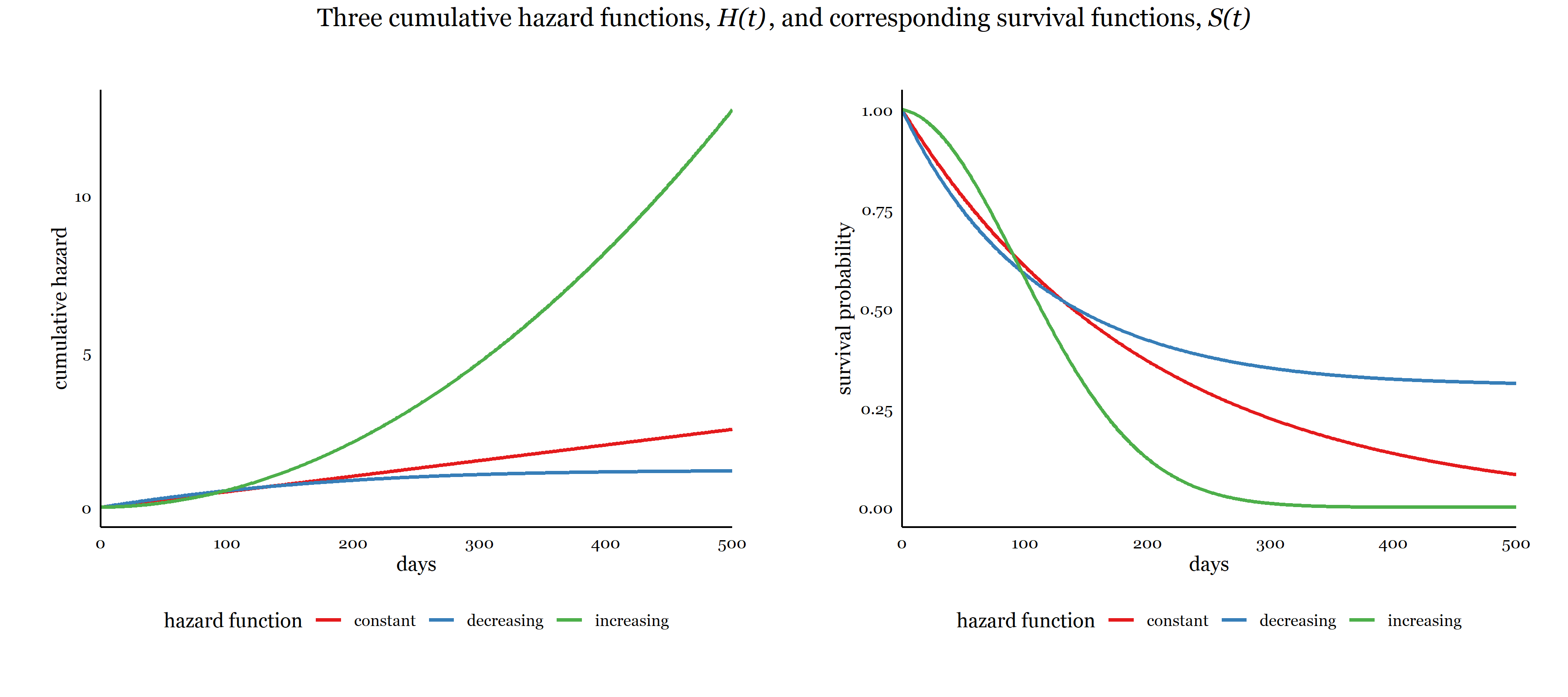Therefore, by modeling either the survival function or the hazard function, we can infer the other.

# Censoring

Many times the exact time when the event is unknown or censored.

Right censoring means that a subject’s actual survival time is greater than their observed time

• study ends before event occurs
• subject is lost to follow-up
• subject is no longer is “at risk” for event after study begins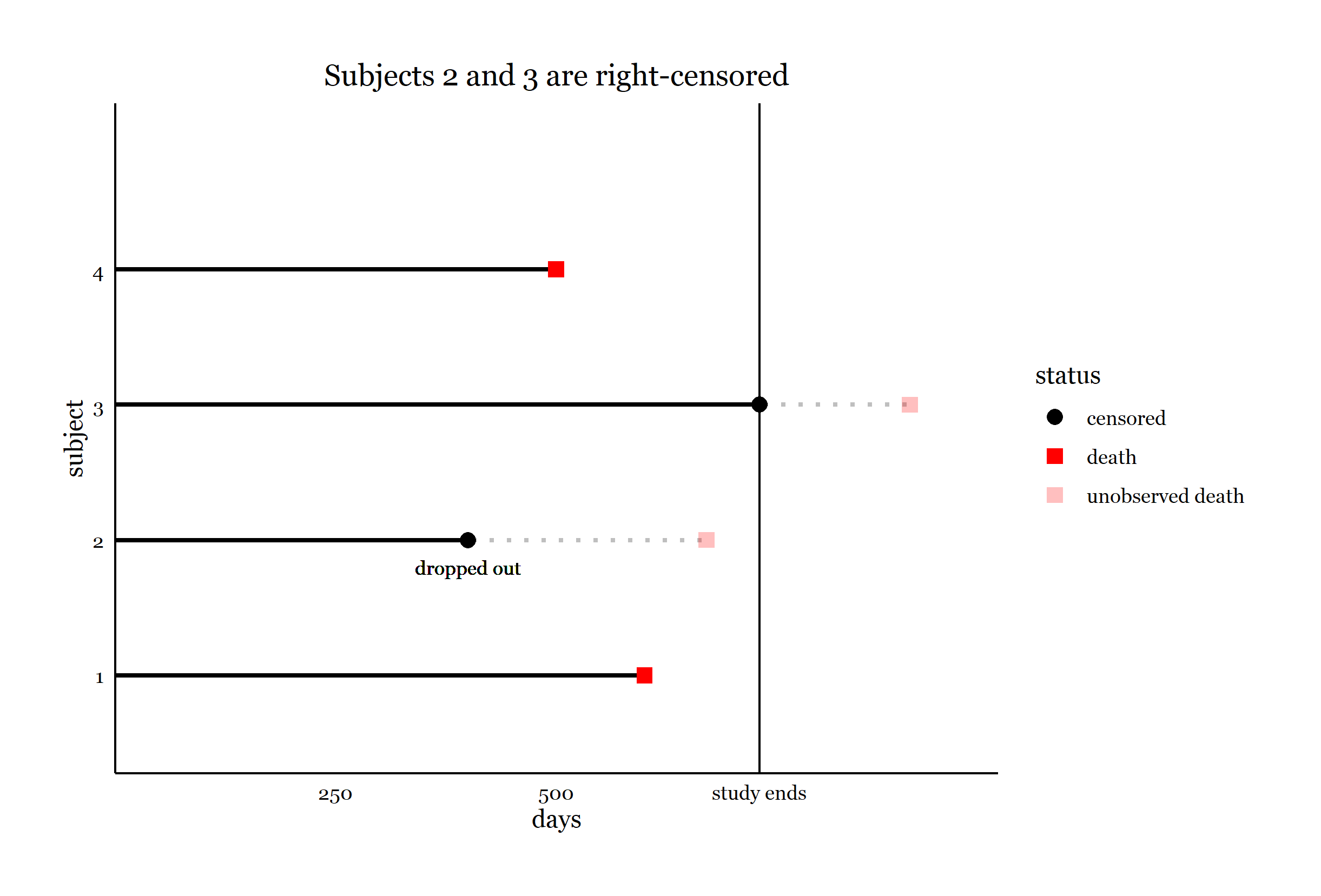Left-censoring means that a subjects actual survival time is less than their observed time. One common example is when the event is defined as disease infection: positive tests for the infection may be delayed by days or even years.

Interval censoring means that a subjects is survival time is unknown, but known to lie between 2 observed time points.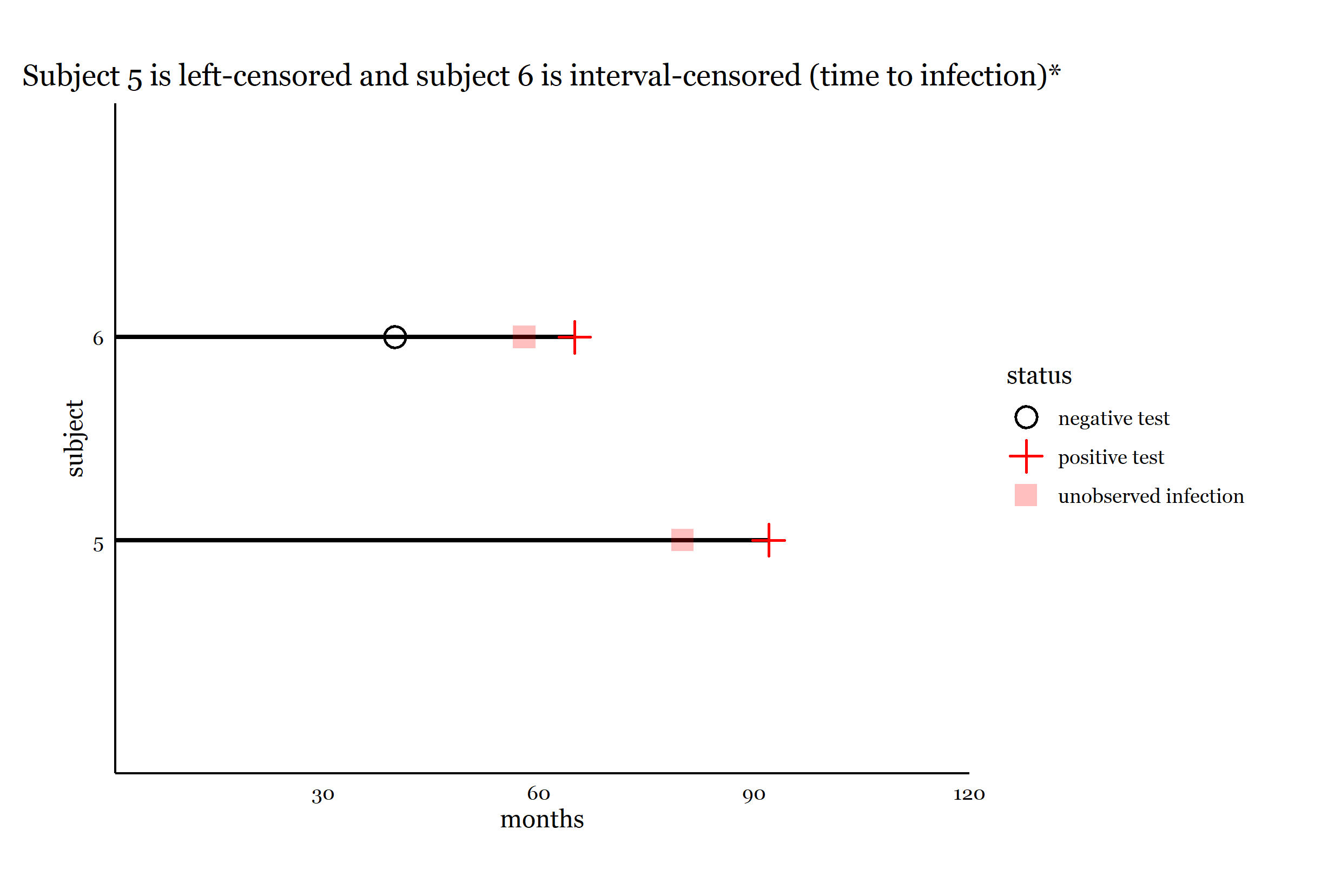We will only discuss methods that handle right-censoring in this workshop.

Many standard methods, such as linear regression, are not equipped to deal with censored outcomes.

*Image adapted from Kleinbaum and Klein, Survival Analysis: A Self-Learning Text, Third Edition, Springer, 2012.

# Assumption of noninformative censoring

Most survival analysis methods, including all those discussed here, assume non-informative censoring.

• a subject’s censoring time should not be related to the unobserved survival time
• distribution of censoring times and survival times are unrelated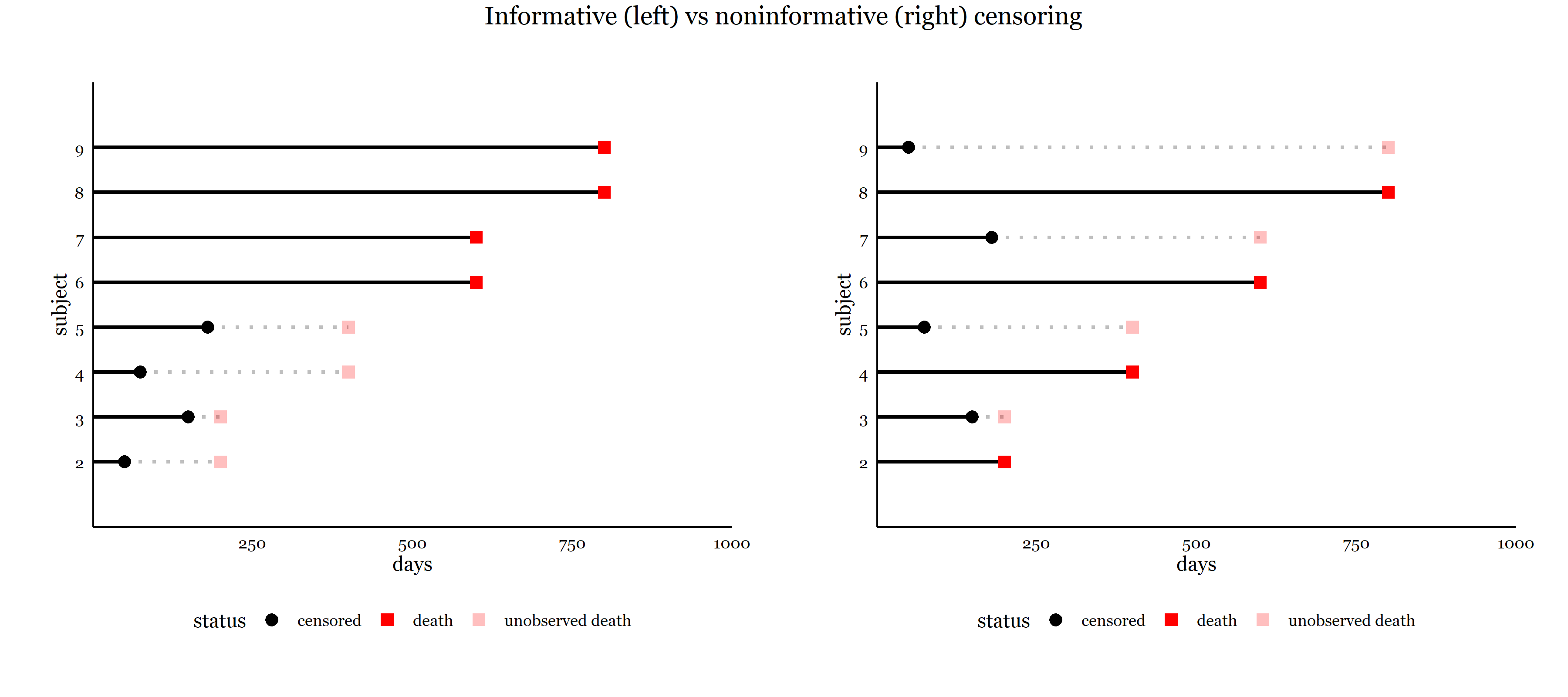Failing to account for informative censoring may result in biased estimates of survival. Below are plots of the Kaplan-Meier survival function estimates of the above data: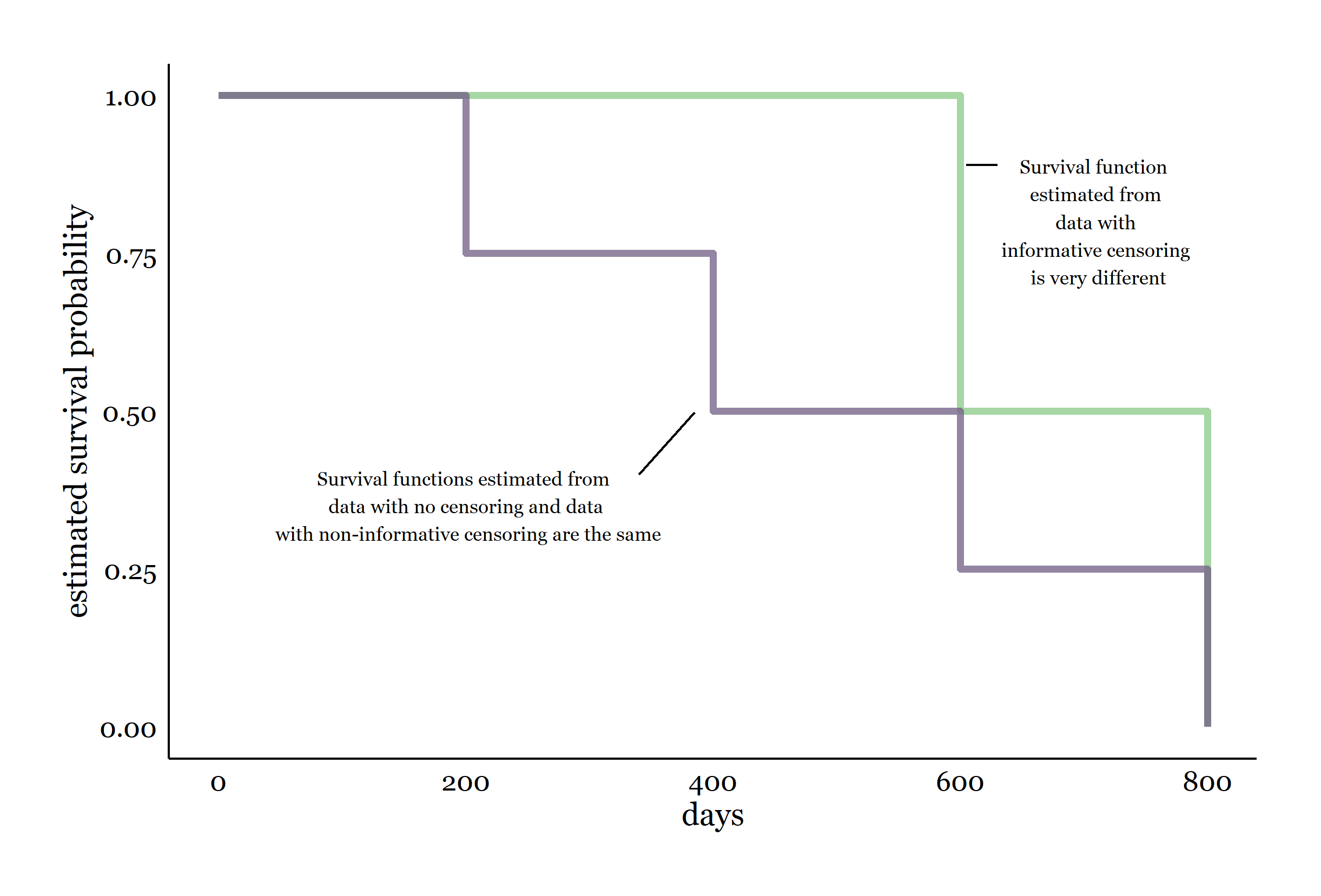Examples of possible informative censoring and resulting bias if not addressed:

• oldest subjects drop out of study of time to death after surgery
• Oldest might have shortest survival times, so survival estimates might be biased upward
• Travel-loving subjects drop out of study of time to first marriage
• Travel-loving subjects may delay marriage to travel more and have longer survival times, so survival estimates might be biased downward

# Data for survival analysis

The simplest data structure for a typical survival analysis is:

• single row per subject
• a status variable coding whether the subject experienced the event or not (censored)
• single time variable measuring $$T$$ time to event (or censoring time, time of last observation)
• variables for covariates, assumed to be time-constant in this structure

# The aml dataset

We’ll start with the aml dataset in the survival package.

These data come from a study looking at time to death for patients with acute myelogenous leukemia, comparing “maintained” chemotherapy treatment to “nonmaintained”.

Variables:

• time  survival or censoring time
• status  0=censored, 1=death
• x  chemotherapy “maintained” or “nonmaintained”
time status x
9 1 Maintained
13 1 Maintained
13 0 Maintained
18 1 Maintained
23 1 Maintained
28 0 Maintained
31 1 Maintained
34 1 Maintained
45 0 Maintained
48 1 Maintained
161 0 Maintained
5 1 Nonmaintained
5 1 Nonmaintained
8 1 Nonmaintained
8 1 Nonmaintained
12 1 Nonmaintained
16 0 Nonmaintained
23 1 Nonmaintained
27 1 Nonmaintained
30 1 Nonmaintained
33 1 Nonmaintained
43 1 Nonmaintained
45 1 Nonmaintained

# The Surv() function for survival outcomes

Use Surv() to specify the survival outcome variables.

Allows for many different time and event status configurations.

For data with a single time variable indicating time to event or censoring, the Surv specification will be:

Surv(time, event)

• time  survival/censoring time variable
• event  status variable. To code for censored/event use:
• 0/1
• 1/2
• FALSE/TRUE

Censoring is assumed to be right-censored unless otherwise specified with the type argument.

# Surv() specification for start-stop format

Some survival analyses require time to be recorded in 2 variables that mark the beginning and end of time intervals. We need this format to model:

• time-varying covariates
• interval censoring
• recurrent events data

In this format, some or all subjects may have multiple rows of data.

This format is sometimes called start-stop format.

The jasa1 data set has this setup, where start and stop are the time variables, and event is the status variable:

head(jasa1)
##     id start stop event transplant        age      year surgery
## 1    1     0   49     1          0 -17.155373 0.1232033       0
## 2    2     0    5     1          0   3.835729 0.2546201       0
## 102  3     0   15     1          1   6.297057 0.2655715       0
## 3    4     0   35     0          0  -7.737166 0.4900753       0
## 103  4    35   38     1          1  -7.737166 0.4900753       0
## 4    5     0   17     1          0 -27.214237 0.6078029       0

To specify the outcome for data in stop-start format, use:

Surv(time, time2, event)

• time and time2  beginning and end of time intervals
• event  is the status at the end of the interval.

# Formula for Kaplan-Meier estimator

The Kaplan-Meier (KM) estimator is a very popular non-parametric method to estimate the survival function, $$S(t)$$. Non-parametric means that we are not assuming any particular distribution for the survival times.

It has an intuitive formula:

$\hat{S}(t)=\prod_{t_i<t}\left(1-\frac{events_i}{num.at.risk_i}\right)$ The expression $$\left(1-\frac{events_i}{num.at.risk_i}\right)$$ is simply the proportion of those at risk that survive time point $$t_i$$. The KM estimator is the product of the proportion that survive each time point $$t_i$$ up to the current time point $$t$$.

Survival estimates do not change if someone drops due to censoring, although the number at risk will drop.

# Kaplan-Meier estimation with survfit()

We can obtain the KM estimate of $$S(t)$$ using survfit().

The first argument is a model formula with a Surv() outcome specification on the left side of ~.

To estimate the survival function for the entire data set, we specify 1 after ~.

# ~ 1 indicates KM survival function estimate for whole sample
KM <- survfit(Surv(time, status) ~ 1, data=aml)

Printing the survfit object gives a summary:

• n: total number at risk
• events: total number of events that occurred
• median: survival time $$t$$ at which $$S(t)=.5$$, or the time at which 50% of those at risk are expected to still be alive
• 0.95LCL, 0.95UCL: 95% confidence limits for median survival
print(KM) 
## Call: survfit(formula = Surv(time, status) ~ 1, data = aml)
##
##       n events median 0.95LCL 0.95UCL
## [1,] 23     18     27      18      45
# same as print(KM)
KM
## Call: survfit(formula = Surv(time, status) ~ 1, data = aml)
##
##       n events median 0.95LCL 0.95UCL
## [1,] 23     18     27      18      45

# Table of KM survival function

The tidy() function from the broom package works with many of the output objects created by the survival package to create tables stored as tibbles (modern data.frames)

Using tidy() on the survfit() object produces a table of the KM estimate of the survival function $$S(t)$$

• time  event/censoring times in the data set
• n.risk, n.event, n.censor  number at risk, number of events, number censored
• estimate  $$\hat{S}(t)$$ survival estimate
• $$\hat{S}(5) = \left(1-\frac{2}{23}\right) = .913$$
• $$\hat{S}(8) = \left(1-\frac{2}{23}\right)\left(1-\frac{2}{21}\right) = .826$$
• std.error, conf.high, conf.low  standard error for $$\hat{S}(t)$$, and 95% confidence interval for $$S(t)$$
# save KM survival function as tibble (modern data.frame)
KM.tab <- tidy(KM)
KM.tab # same as print(KM.tab)
## # A tibble: 18 × 8
##     time n.risk n.event n.censor estimate std.error conf.high conf.low
##    <dbl>  <dbl>   <dbl>    <dbl>    <dbl>     <dbl>     <dbl>    <dbl>
##  1     5     23       2        0   0.913     0.0643     1       0.805
##  2     8     21       2        0   0.826     0.0957     0.996   0.685
##  3     9     19       1        0   0.783     0.110      0.971   0.631
##  4    12     18       1        0   0.739     0.124      0.942   0.580
##  5    13     17       1        1   0.696     0.138      0.912   0.531
##  6    16     15       0        1   0.696     0.138      0.912   0.531
##  7    18     14       1        0   0.646     0.157      0.878   0.475
##  8    23     13       2        0   0.547     0.196      0.803   0.372
##  9    27     11       1        0   0.497     0.218      0.762   0.324
## 10    28     10       0        1   0.497     0.218      0.762   0.324
## 11    30      9       1        0   0.442     0.248      0.718   0.272
## 12    31      8       1        0   0.386     0.282      0.671   0.223
## 13    33      7       1        0   0.331     0.321      0.622   0.177
## 14    34      6       1        0   0.276     0.369      0.569   0.134
## 15    43      5       1        0   0.221     0.432      0.515   0.0947
## 16    45      4       1        1   0.166     0.519      0.458   0.0598
## 17    48      2       1        0   0.0828    0.877      0.462   0.0148
## 18   161      1       0        1   0.0828    0.877      0.462   0.0148

# Graphing the survival function

We can plot the KM survival function and confidence intervals for the entire aml sample.

Notice how the KM-estimated $$\hat{S}(t)$$ is a step function, where $$\hat{S}(t)$$ only changes at timepoints when an event occurs.

The true underlying survival curve $$S(t)$$ may be smooth, but the smoothness of the KM curve is limited by the number of event times observed in the data.

plot(KM, ylab="survival probability", xlab="months")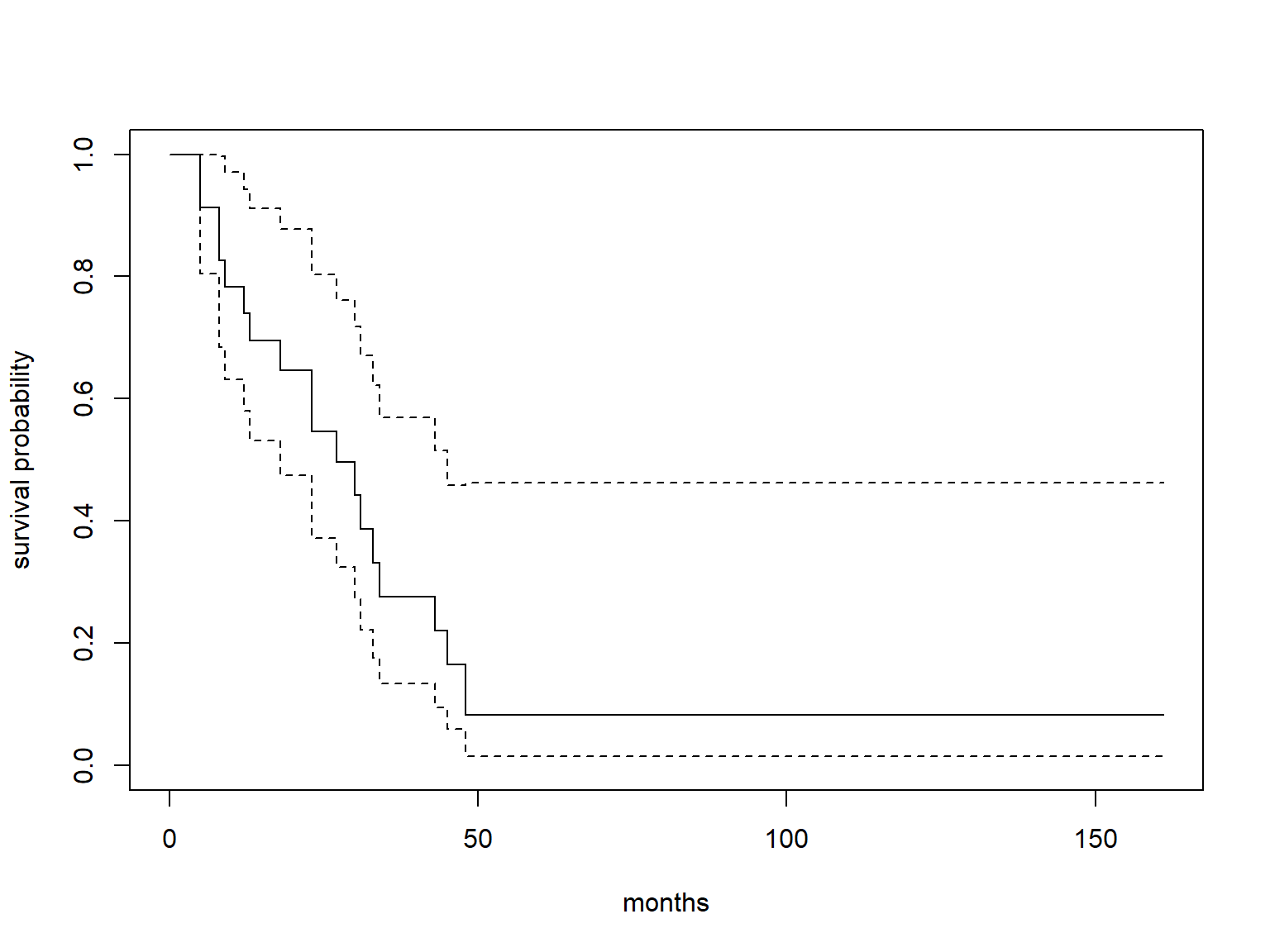# Stratified Kaplan-Meier estimates

To estimate separate KM survival functions for different strata, specify one or more strata variables after the ~ in survfit()

# stratify by x variable
KM.x <- survfit(Surv(time, status) ~ x, data=aml)

# median survival by strata
KM.x
## Call: survfit(formula = Surv(time, status) ~ x, data = aml)
##
##                  n events median 0.95LCL 0.95UCL
## x=Maintained    11      7     31      18      NA
## x=Nonmaintained 12     11     23       8      NA

Notice the addition of the strata column in the tidy() output:

# KM estimated survival functions by strata
tidy(KM.x)
## # A tibble: 20 × 9
##     time n.risk n.event n.censor estimate std.error conf.high conf.low strata
##    <dbl>  <dbl>   <dbl>    <dbl>    <dbl>     <dbl>     <dbl>    <dbl> <chr>
##  1     9     11       1        0   0.909     0.0953     1       0.754  x=Mainta…
##  2    13     10       1        1   0.818     0.142      1       0.619  x=Mainta…
##  3    18      8       1        0   0.716     0.195      1       0.488  x=Mainta…
##  4    23      7       1        0   0.614     0.249      0.999   0.377  x=Mainta…
##  5    28      6       0        1   0.614     0.249      0.999   0.377  x=Mainta…
##  6    31      5       1        0   0.491     0.334      0.946   0.255  x=Mainta…
##  7    34      4       1        0   0.368     0.442      0.875   0.155  x=Mainta…
##  8    45      3       0        1   0.368     0.442      0.875   0.155  x=Mainta…
##  9    48      2       1        0   0.184     0.834      0.944   0.0359 x=Mainta…
## 10   161      1       0        1   0.184     0.834      0.944   0.0359 x=Mainta…
## 11     5     12       2        0   0.833     0.129      1       0.647  x=Nonmai…
## 12     8     10       2        0   0.667     0.204      0.995   0.447  x=Nonmai…
## 13    12      8       1        0   0.583     0.244      0.941   0.362  x=Nonmai…
## 14    16      7       0        1   0.583     0.244      0.941   0.362  x=Nonmai…
## 15    23      6       1        0   0.486     0.305      0.883   0.268  x=Nonmai…
## 16    27      5       1        0   0.389     0.378      0.816   0.185  x=Nonmai…
## 17    30      4       1        0   0.292     0.476      0.741   0.115  x=Nonmai…
## 18    33      3       1        0   0.194     0.627      0.664   0.0569 x=Nonmai…
## 19    43      2       1        0   0.0972    0.945      0.620   0.0153 x=Nonmai…
## 20    45      1       1        0   0       Inf         NA      NA      x=Nonmai…

# Graphing stratified KM estimates of survival

plot() will only produce confidence intervals for $$S(t)$$ by default if one curve is plotted.$$\dagger$$

For multiple curves, we must request confidence intervals with conf.int=T.

We also specify two colors to make the graph more readable.

# stratified KM curves with 95% CI, 2 colors
plot(KM.x, ylab="survival probability", xlab="months",
conf.int=T, col=c("red", "blue"))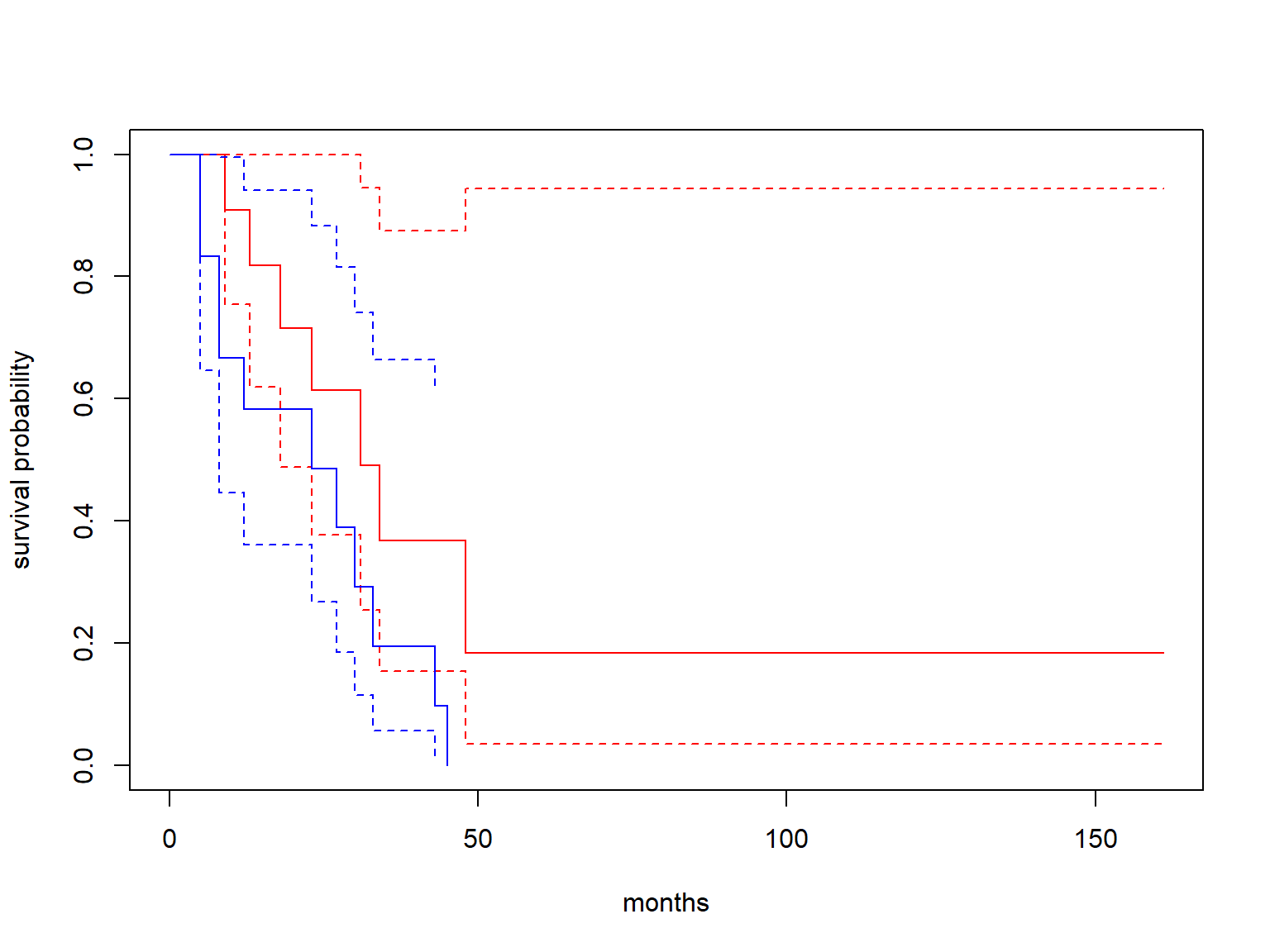$$\dagger$$ plot() is a generic function and calls plot.survfit() when supplied a survfit object. The option conf.int= is an option of plot.survfit().

# Customizable, informative survival plots with survminer

plot.survfit() uses base R graphics and has limited options to customize a plot of survival functions.

The survminer package leverages the graphical power of the ggplot2 package and adds many of its own features to create highly customizable plots of survival functions.

Using ggsurvplot() from survminer on a survfit object produces a plot of the KM estimated survival functions.

ggsurvplot(KM.x, conf.int=T)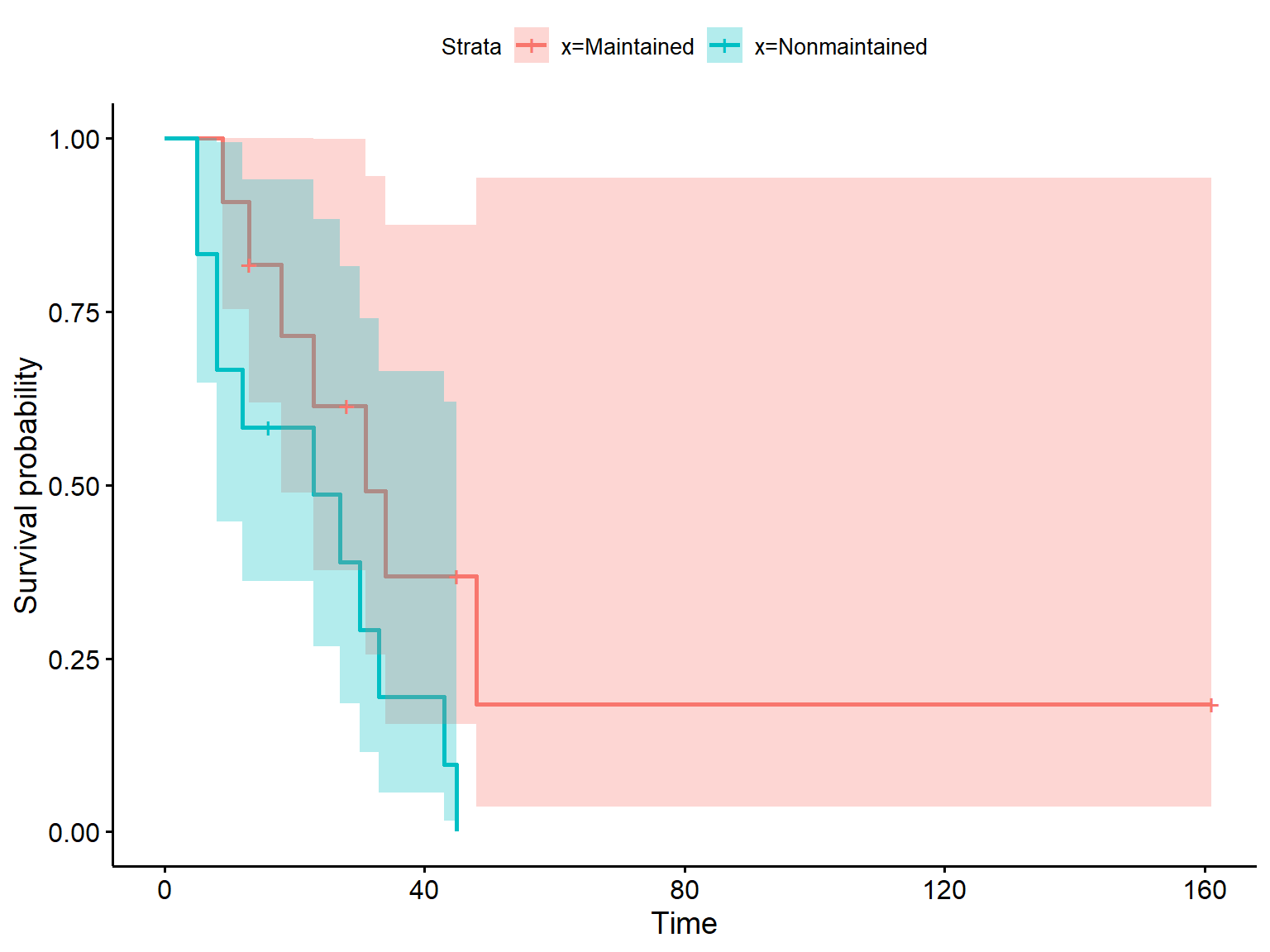Notice that ggsurvplot() automatically adds + symbols to denote censored observations.

ggsurvplot() makes adding a risk table very easy.

ggsurvplot(KM.x, conf.int=T,
risk.table=T)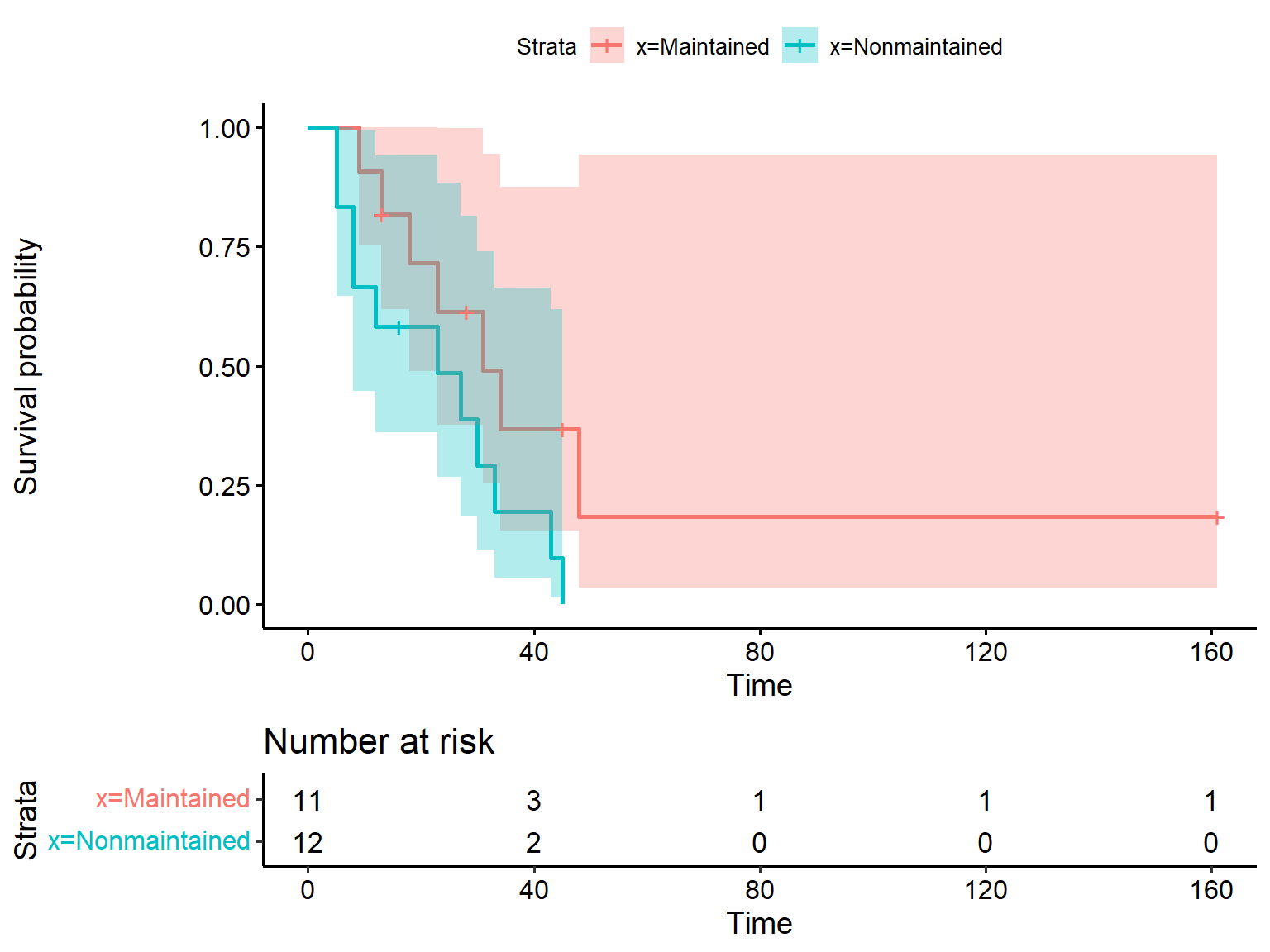You can pass most arguments to various functions in ggplot2 through ggsurvplot().

ggsurvplot(KM.x, conf.int=T,
risk.table=T,
palette="Accent", # argument to scale_color_brewer()
size=2, # argument to geom_line()
ggtheme = theme_minimal()) # changing ggtheme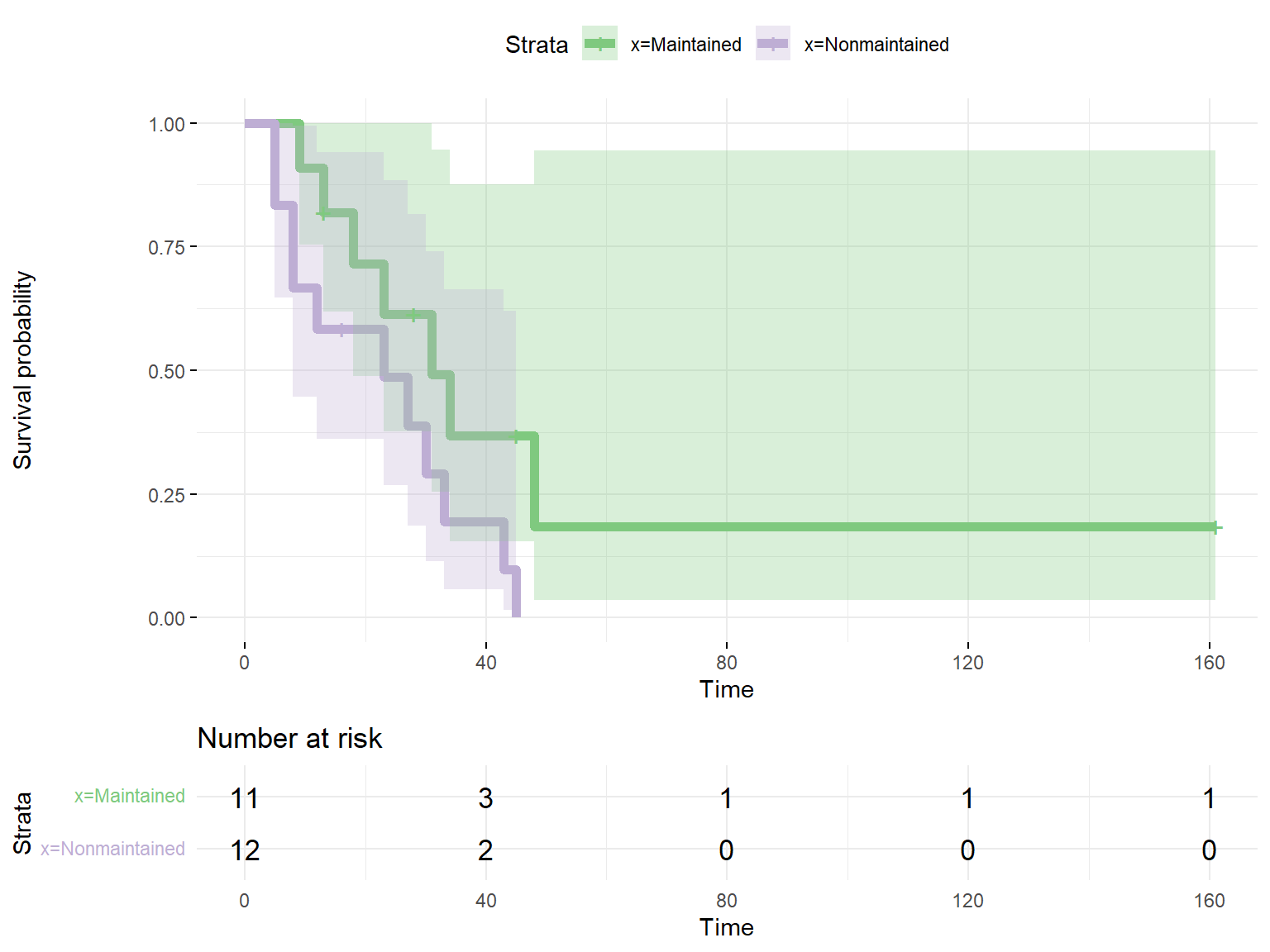You can also use traditional ggplot2 syntax by extracting the ggplot object as ggsurvplot()$plot. g <- ggsurvplot(KM.x, conf.int=T, risk.table=T)$plot  # this is the ggplot object
g + scale_fill_grey() + scale_color_grey()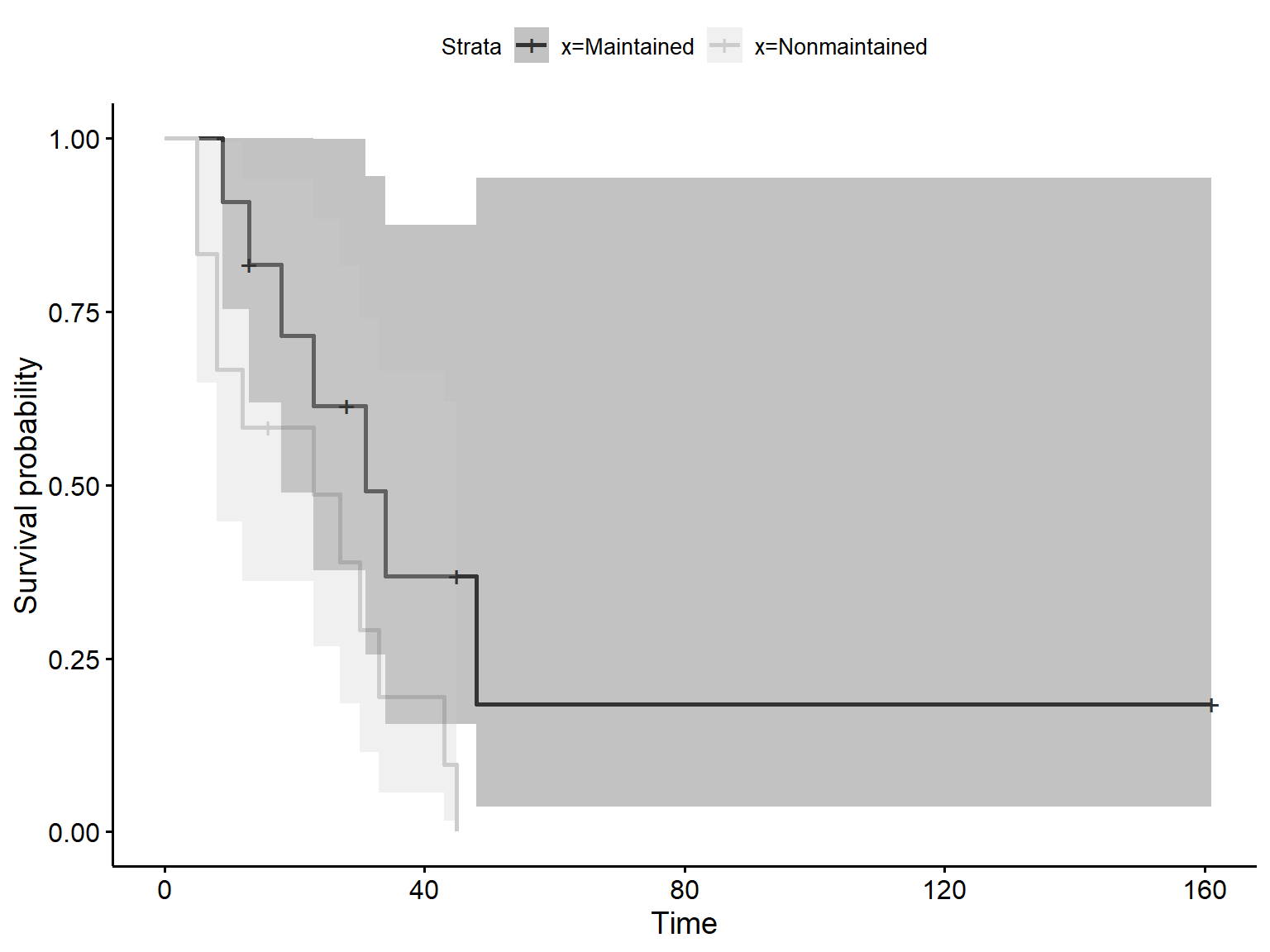See our ggplot2 seminar to learn how to use the ggplot2 package.

# Comparing survival functions with survdiff()

We often want to test the hypothesis:

$$H_0$$: survival curves across 2 or more groups are equivalent
$$H_A$$: survival curves across 2 or more groups are not equivalent

The log-rank statistic is one popular method to evaluate this hypothesis.

Under the null, the log-rank statistic is $$\chi^2$$ distributed with $$g-1$$ degrees of freedom.

The function survdiff() performs the log-rank test by default.

# log rank test, default is rho=0
survdiff(Surv(time, status) ~ x, data=aml)
## Call:
## survdiff(formula = Surv(time, status) ~ x, data = aml)
##
##                  N Observed Expected (O-E)^2/E (O-E)^2/V
## x=Maintained    11        7    10.69      1.27       3.4
## x=Nonmaintained 12       11     7.31      1.86       3.4
##
##  Chisq= 3.4  on 1 degrees of freedom, p= 0.07

We see some evidence, not strong, that the survival curves may be different.

survdiff() allows weights in calculation of the $$\chi^2$$ statistic with the rho= argument. The weights are defined as $$\hat{S}(t)^\rho$$, where $$0 \le \rho \le 1$$.

• rho=0  equal weights, $$\hat{S}(t)^0=1$$, which is the log-rank test and the default
• rho=1  the weights equal the survival estimate itself, $$\hat{S}(t)^1=\hat{S}(t)$$, equivalent to the Gehan-Wilcoxon test
• earlier timepoints are weighted more heavily (might make sense for death after surgery, for example)
• rho=  values between 0 and 1 are valid, with values closer to 1 putting more weight on earlier time points
# rho=1 specifies Peto & Peto modification of Gehan-Wilcoxon,
#   more weight put on earlier time points
survdiff(Surv(time, status) ~ x, data=aml, rho=1)
## Call:
## survdiff(formula = Surv(time, status) ~ x, data = aml, rho = 1)
##
##                  N Observed Expected (O-E)^2/E (O-E)^2/V
## x=Maintained    11     3.85     6.14     0.859      2.78
## x=Nonmaintained 12     7.18     4.88     1.081      2.78
##
##  Chisq= 2.8  on 1 degrees of freedom, p= 0.1

# Exercise 1

The veteran data set describes survival times for veterans with lung cancer.

Variables:

• time: survival time
• status: status, 0=censored, 1=dead
• trt: treatment, 1=standard, 2=test

1. Create a graph and table of the Kaplan-Meier estimated survival function for the entire data set. What is the median survival time?
2. Create a graph of KM estimated survival functions stratified by treatment, adding 95% confidence intervals and coloring the 2 functions. Does survival appear different for the 2 treatment groups?
3. Use the log-rank test to provide more evidence for your assessment of survival of the 2 groups.

# Background

Instead of estimating the survival function $$S(t)$$ directly, the Cox proportional hazards model estimates changes to the hazard function, $$h(t)$$.

The Cox model can estimate the effects of multiple predictors$$^\dagger$$ on the hazard function.

By far the most popular method for survival analysis

• no distribution assumed for survival times
• naturally accommodates right-censoring and time-varying covariates
• can be extended in many ways:
• time-varying coefficients
• random effect frailties for recurrent events or clustering
• competing risks modeling

$$^\dagger$$We use the words predictor and covariate interchangeably throughout this workshop.

# The Cox proportional hazards model

For simplicity, we begin with a Cox model with a single time-constant predictor, $$X_1$$:

$h(t|X_1=x_1) = h_0(t)exp(b_1x_1)$

• $$h(t|X_1=x_1)$$: the hazard at time $$t$$ for a subject with predictor $$X_1$$ equal to the value $$x_1$$
• $$h_0(t)$$: the baseline hazard at time $$t$$, the hazard for a subject with all predictors equal to zero
• $$exp(b_1x_1)$$: the hazard ratio comparing the hazard for a subject with $$X_1=x_1$$ to a subject with $$X_1=0$$

# Hazard ratio

For example, imagine that $$X_1$$ is a treatment variable, with values $$X_1=1$$ for treatment and $$X_1=0$$ for control.

The hazard at time $$t$$ for treatment:

\begin{aligned}h(t|X_1=1) &= h_0(t)exp(b_1*1)\\ &= h_0(t)exp(b_1) \end{aligned}

and for control:

\begin{aligned}h(t|X_1=0) &= h_0(t)exp(b_1*0)\\ &= h_0(t)exp(0) \\ &= h_0(t) \end{aligned}

We can compare the hazards for treatment and control at time $$t$$ as a hazard ratio (HR):

\begin{aligned}HR&=\frac{h(t|X_1=1)}{h(t|X_1=0)} \\ &= \frac{h_0(t)exp(b_1)}{h_0(t)} \\ &= exp(b_1) \end{aligned} Thus, $$exp(b_1)$$ is the hazard ratio comparing the hazard for treatment to controls.

• $$HR=.25$$ means that treatment has $$\frac{1}{4}$$ (25%) the hazard of control, or a 75% decrease. With a lower hazard rate, treatment will have fewer expected events and thus better survival.
• $$HR=2$$ here means that treatment has twice the hazard of control, or a 100% increase, and thus worse survival.

In general, $$exp(b_1)$$ expresses the hazard ratio for a 1-unit increase in the associated covariate.

$$b_1$$ itself is the log-hazard ratio.

# Proportional hazards

The standard Cox model assumes proportional hazards, which means that the effects of covariates are constant over time.

For example, in our simple Cox model for a treatment effect, proportional hazards means that the effect of treatment does not change over time.

Notice that the expression for the hazard ratio for the treatment effect does not contain time, so it will be the same value no matter the time:

$HR=exp(b_1)$

Note: With proportional hazards, a subject’s hazard function (which we don’t need to know for the Cox model) can change over time. But the hazard ratio comparing that subject’s hazard to another subject’s hazard cannot change over time, provided their covariate values do not change.

Visually, this is represented by “parallel” survival curves that should not cross: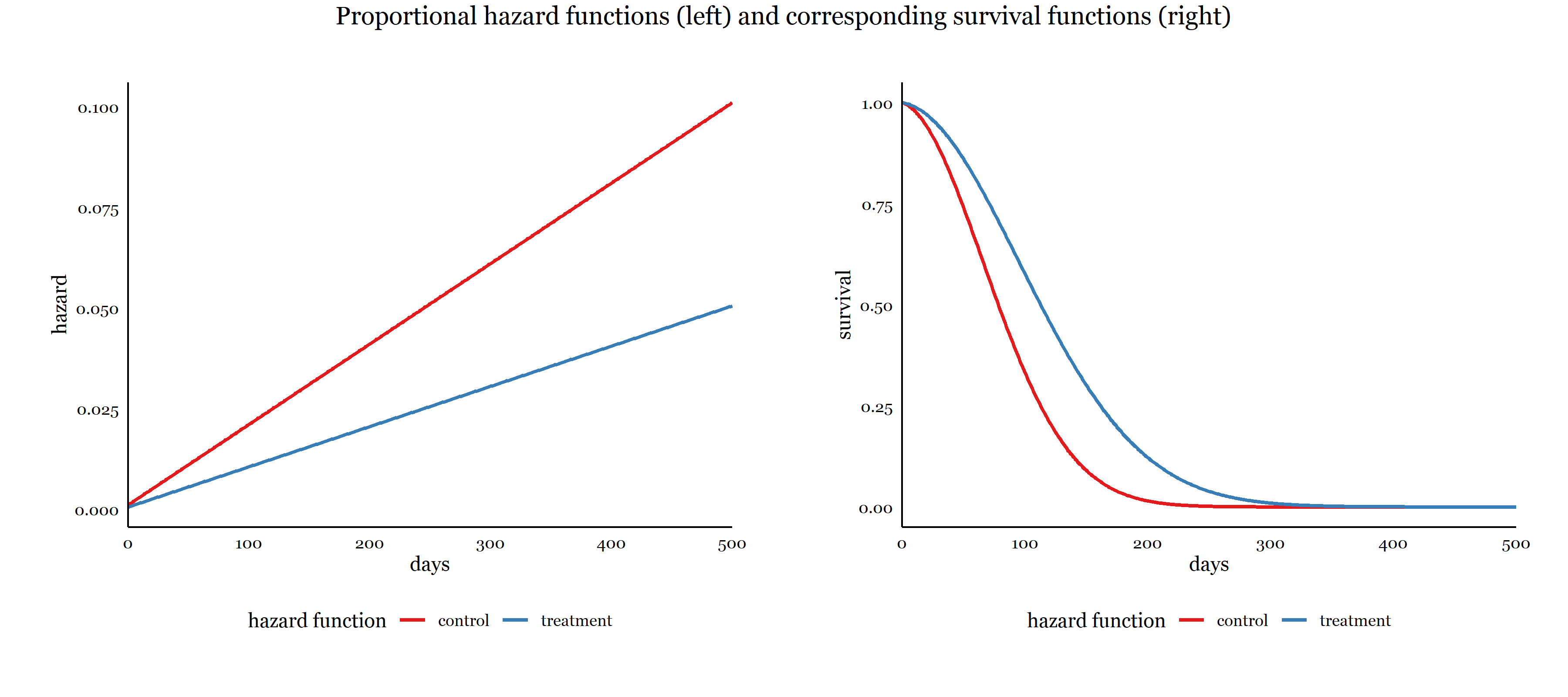The parallelism is easier to evaluate if we plot $$-log(-log(S(t)))$$, the negative log of the negative log of the survival function. If the hazards are proportional, the vertical distance between the curves is constant across time.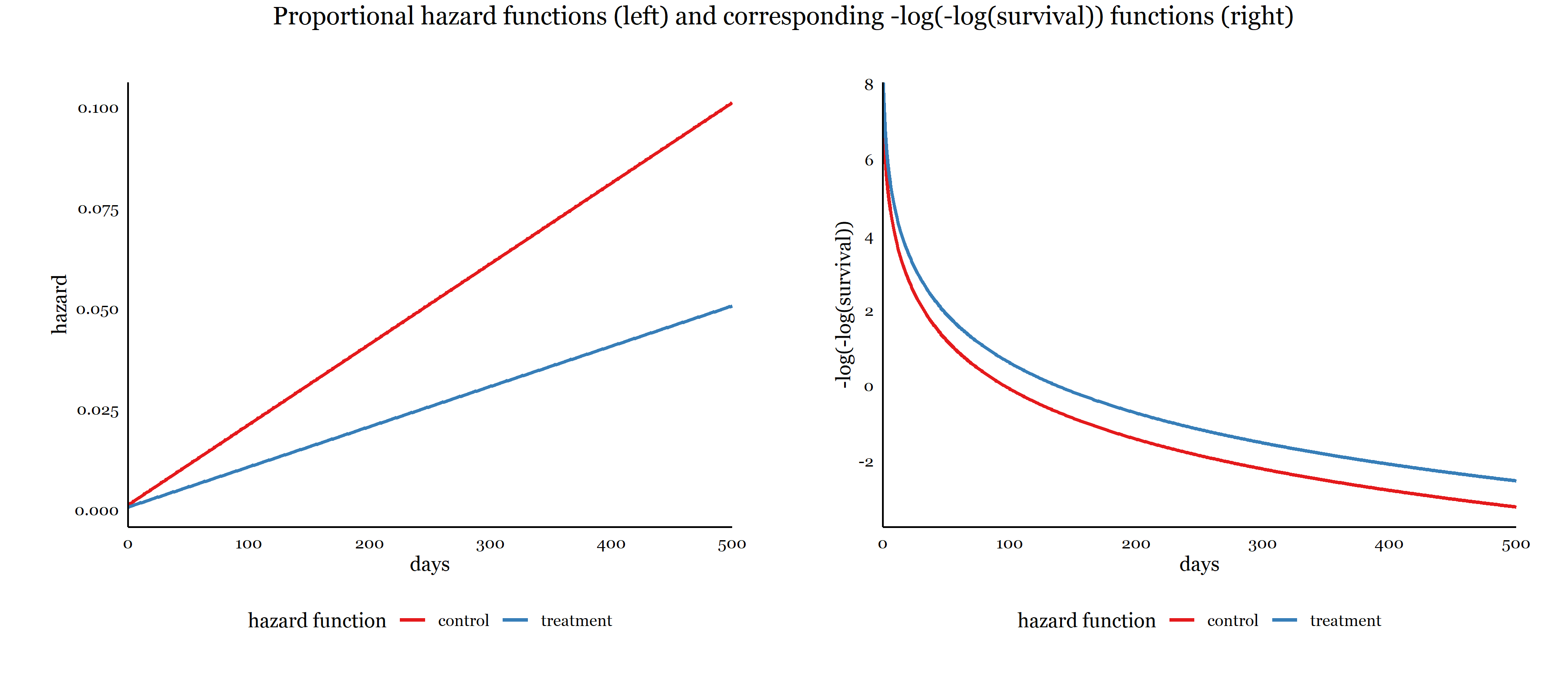Violation of proportional hazards suggests that a predictor’s effect changes over time.

Hazard and survival functions will often cross: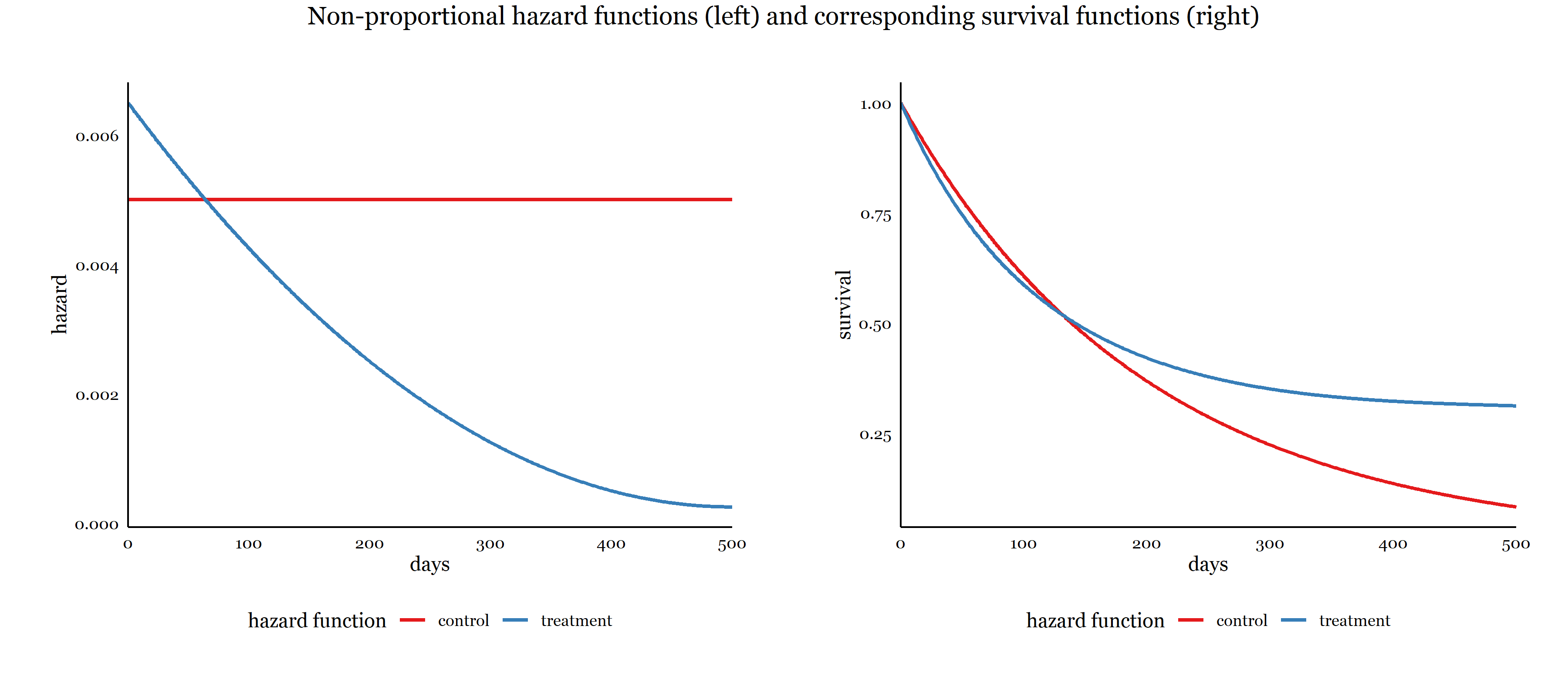As will graphs of $$-log(-log(S(t)))$$, where we see that the vertical distance between curves changes and reverses direction over time: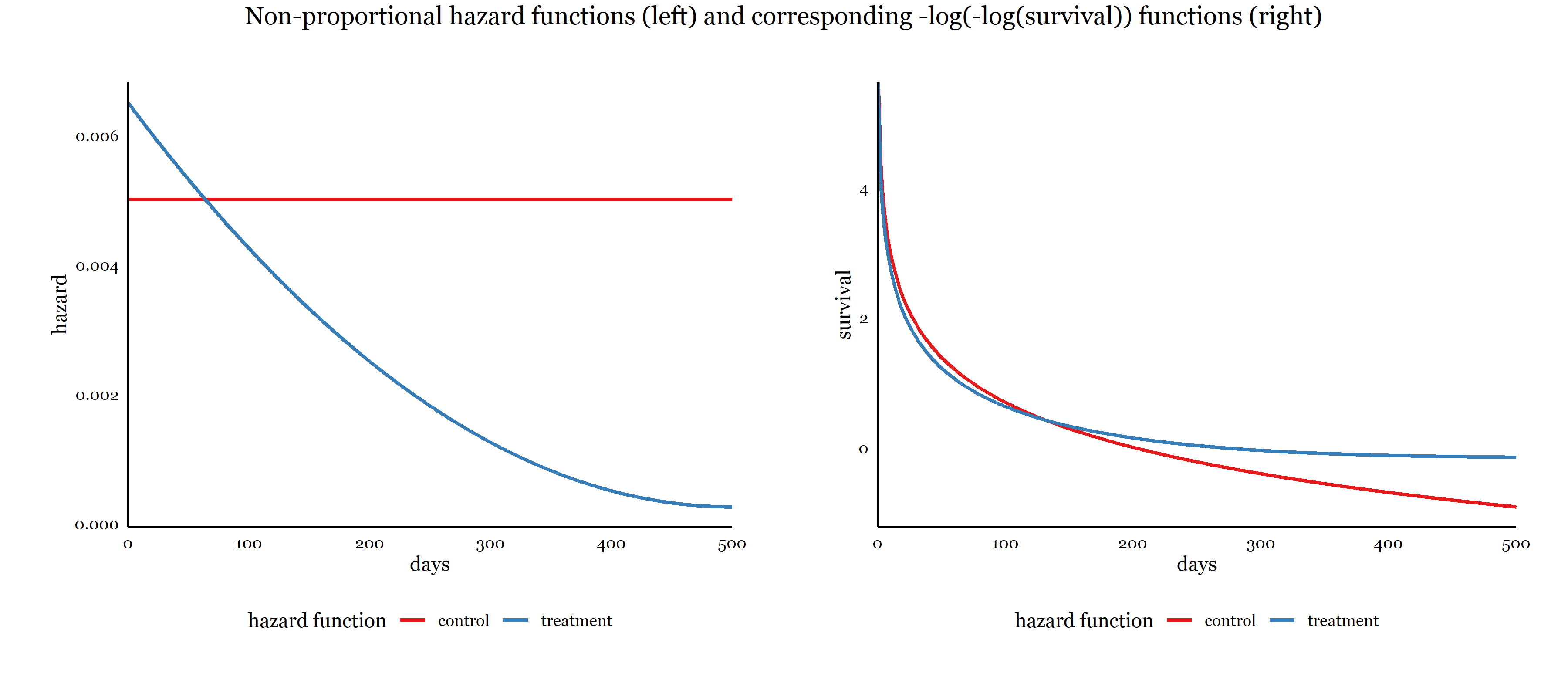Failing to account for non-constant hazard ratios threatens validity of Cox model estimates.

The Cox model can be extended in various ways to accommodate non-proportional hazards.

# Baseline hazard function $$h_0(t)$$

One reason why the Cox model is so popular is that it does not require specification of the baseline hazard function, $$h_0(t)$$, the hazard function for a subject with zero on all covariates.

Essentially, this means we can use the Cox model without assuming a particular form of the hazard function or assuming a distribution of survival times.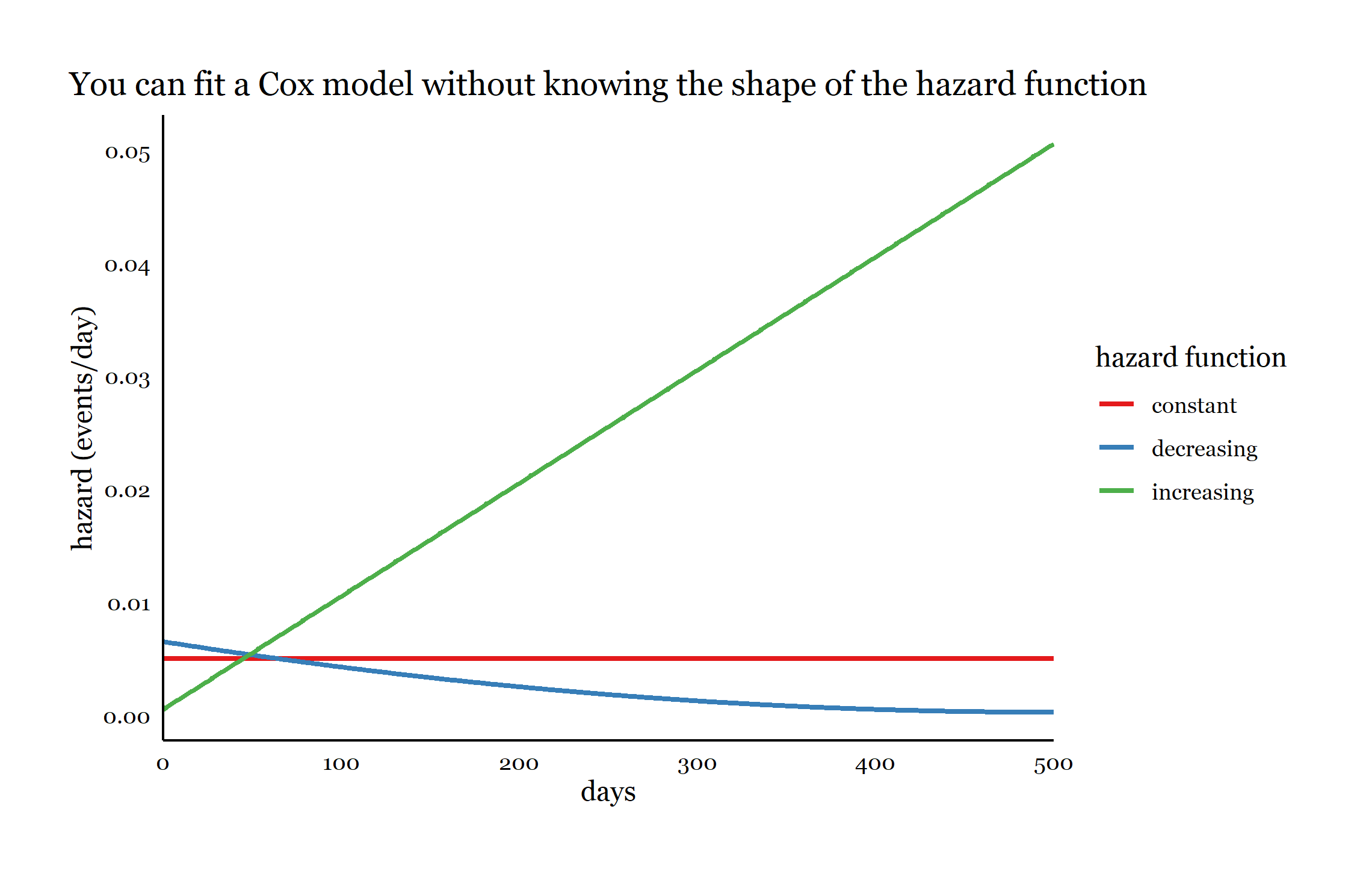We thus then do not need to estimate parameters to characterize a hazard function or survival distribution, but only the regression parameters that quantify the covariate effects. The Cox model is thus called semiparametric.

No constant/intercept in Cox models.

Because $$h_0(t)$$ is left unspecified, the Cox model can not directly estimate either the hazard function or the survival function, but is used to estimate covariate effects on the hazard functions.

# Cox model with multiple predictors

The Cox is easily extended to accommodate multiple predictors, each of whose effects is assumed to be proportional over time.

$h(t|X_1, X_2, ...X_p) = h_0(t)exp(b_1X_1 + b_2X_2 + ... + b_pX_p)$ Each coefficient $$b_i$$ can be exponentiated to calculate a hazard ratio.

# New data set for Cox modeling

We will be using the lung data set form the survival package for Cox modeling.

The data describe survival of patients with advanced lung cancer.

Variables:

• time: survival time in days
• status: 1=censored, 2=dead$$^\dagger$$
• age: age in years (assessed at beginning)
• sex: 1=male, 2=female$$^\ddagger$$
• wt.loss: weight loss (pounds) in last 6 months

$$^\dagger$$Remember that the Surv() function accepts a status variable with 1=censored and 2=event

$$^\ddagger$$We would normally recommend that binary variables be coded 0/1 so that the intercept is interpretable; however, there is no intercept in the Cox model, so 1/2 coding is equivalent.

# Fitting a Cox model

Use coxph(formula,data=)

THe formula resembles a typical R regression formula:

Surv(time, event) ~ x1 + x2…

where x1 + x2… is a list of one or more predictor variables separated by +.

# fit cox model and save results
lung.cox <- coxph(Surv(time, status) ~ age + sex + wt.loss, data=lung)
# summary of results
summary(lung.cox)
## Call:
## coxph(formula = Surv(time, status) ~ age + sex + wt.loss, data = lung)
##
##   n= 214, number of events= 152
##    (14 observations deleted due to missingness)
##
##               coef  exp(coef)   se(coef)      z Pr(>|z|)
## age      0.0200882  1.0202913  0.0096644  2.079   0.0377 *
## sex     -0.5210319  0.5939074  0.1743541 -2.988   0.0028 **
## wt.loss  0.0007596  1.0007599  0.0061934  0.123   0.9024
## ---
## Signif. codes:  0 '***' 0.001 '**' 0.01 '*' 0.05 '.' 0.1 ' ' 1
##
##         exp(coef) exp(-coef) lower .95 upper .95
## age        1.0203     0.9801    1.0011    1.0398
## sex        0.5939     1.6838    0.4220    0.8359
## wt.loss    1.0008     0.9992    0.9887    1.0130
##
## Concordance= 0.612  (se = 0.027 )
## Likelihood ratio test= 14.67  on 3 df,   p=0.002
## Wald test            = 13.98  on 3 df,   p=0.003
## Score (logrank) test = 14.24  on 3 df,   p=0.003

Notice:

• sample size (214), number of events (152), number of observations dropped due to missingness
• coef: log hazard ratio coefficients
• generally, we interpret positive coefficients as increasing the log-hazard and lowering survival, and negative coefficients as decreasing the log-hazard and increasing survival
• exp(coef): hazard ratios (exponentiated coefficients)
• $$\hat{HR}_{age}=1.0203$$, for each additional year of age at baseline, the hazard increases by 2.03%, or by a factor of 1.0203
• $$\hat{HR}_{sex}=.5939$$, females have 60% the hazard of males, or a 40% decrease
• $$\hat{HR}_{wt.loss}=1.008$$, for each additional pound of weight loss, the hazard increases by 0.08%
• se(coef): standard error of log hazard ratios
• Pr(>|z|): p-value for test of log hazard ratio = 0, or equivalently, hazard ratio = 1
• lower .95, upper .95: 95% confidence interval for hazard ratio
• the data are consistent with hazard ratio estimates for sex between .422 and .836
• the confidence interval for wt.loss indicates that we cannot be sure of the direction of its effect, if any
• Concordance: proportion of pairs that are concordant, a goodness-of-fit measure
• Likelihood ratio, Wald, and Score tests: 3 tests of the null hypothesis that all coefficients equal zero

# Tidy coxph() results

We can tidy() the coxph() results and store them in a tibble data.frame.

# save summarized results as data.frame
#  exponentiate=T returns hazard ratios
lung.cox.tab <- tidy(lung.cox, exponentiate=T, conf.int=T)

# display table
lung.cox.tab
## # A tibble: 3 × 7
##   term    estimate std.error statistic p.value conf.low conf.high
##   <chr>      <dbl>     <dbl>     <dbl>   <dbl>    <dbl>     <dbl>
## 1 age        1.02    0.00966     2.08  0.0377     1.00      1.04
## 2 sex        0.594   0.174      -2.99  0.00280    0.422     0.836
## 3 wt.loss    1.00    0.00619     0.123 0.902      0.989     1.01

Storing the Cox model results as a data.frame makes it easy to use ggplot2 to create plots of the hazard ratios and confidence intervals.

# plot of hazard ratios and 95% CIs
ggplot(lung.cox.tab,
aes(y=term, x=estimate, xmin=conf.low, xmax=conf.high)) +
geom_pointrange() +  # plots center point (x) and range (xmin, xmax)
geom_vline(xintercept=1, color="red") + # vertical line at HR=1
labs(x="hazard ratio", title="Hazard ratios and 95% CIs") +
theme_classic()